• +91 9971497814
• info@interviewmaterial.com

# RD Chapter 10- Congruent Triangles Ex-MCQS Interview Questions Answers

### Related Subjects

Question 1 :
One angle is equal to three times its supplement. The measure of the angle is
(a) 130°
(b) 135°
(c) 90°
(d) 120°

Let required angle = x
Then its supplement = (180° – x)
x = 3(180° – x) = 540° – 3x
⇒ x + 3x = 540°
⇒ 4x = 540°
⇒ x =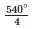= 135°
∴ Required angle = 135° (b)

Question 2 :
Two straight lines AB and CD intersect one another at the point O. If ∠AOC + ∠COB + ∠BOD = 274°, then ∠AOD =
(a) 86°
(b) 90°
(c) 94°
(d) 137°

Sum of angles at a point O = 360°
Sum of three angles ∠AOC + ∠COB + ∠BOD = 274°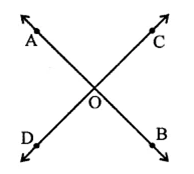∴ Fourth angle ∠AOD = 360° – 274°
= 86° (a)

Question 3 :
Two straight lines AB and CD cut each other at O. If ∠BOD = 63°, then ∠BOC =
(a) 63°
(b) 117°
(c) 17°
(d) 153°

CD is a line
∴ ∠BOD + ∠BOC = 180° (Linear pair)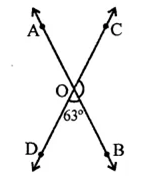⇒ 63° + ∠BOC = 180°
⇒ ∠BOC = 180° – 63°
∴ ∠BOC =117° (b)

Question 4 :
Consider the following statements:
When two straight lines intersect:
(iii) opposite angles are equal
(iv) opposite angles are supplementary Of these statements
(a) (i) and (iii) are correct
(b) (ii) and (iii) are correct
(c) (i) and (iv) are correct
(d) (ii) and (iv) are correct

Answer 4 : Only (ii) and (iii) arc true. (b)

Question 5 :
Given ∠POR = 3x and ∠QOR = 2x + 10°. If POQ is a striaght line, then the value of x is
(a) 30°
(b) 34°
(c) 36°
(d) none of these

∵ POQ is a straight line
∴ ∠POR + ∠QOR = 180° (Linear pair)
⇒ 3x + 2x + 10° = 180°
⇒ 5x = 180 – 10° = 170°
∴ x =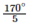= 34° (b)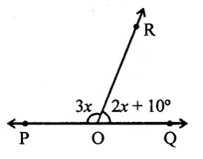Question 6 :
In the figure, AOB is a straight line. If ∠AOC + ∠BOD = 85°, then ∠COD =
(a) 85°
(b) 90°
(c) 95°
(d) 100°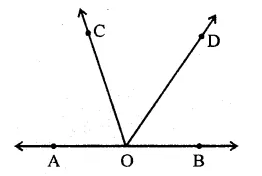AOB is a straight line,
OC and OD are rays on it
and ∠AOC + ∠BOD = 85°
But ∠AOC + ∠BOD + ∠COD = 180°
⇒ 85° + ∠COD = 180°
∠COD = 180° – 85° = 95° (c)

Question 7 :
In the figure, the value of y is
(a) 20°
(b) 30°
(c) 45°
(d) 60°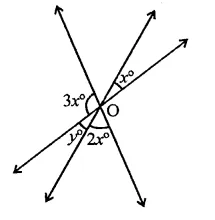In the figure,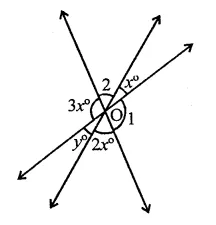y = x (Vertically opposite angles)
∠1 = 3x
∠2 = 3x
∴ 2(x + 3x + 2x) = 360° (Angles at a point)
2x + 6x + 4x = 360°
12x = 360° ⇒ x =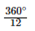= 30°
∴ y = x = 30° (b)

Question 8 :
In the figure, the value of x is
(a) 12
(b) 15
(c) 20
(d) 30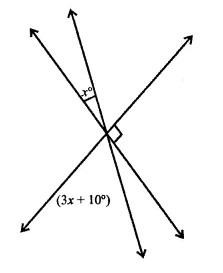∠1 = 3x+ 10 (Vertically opposite angles)
But x + ∠1 + ∠2 = 180°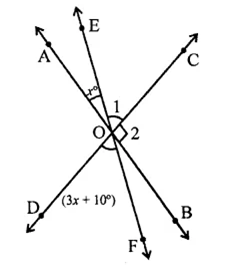⇒ x + 3x + 10° + 90° = 180°
⇒ 4x = 180° – 10° – 90° = 80°
x =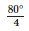= 20   (c)

Question 9 :
In the figure, which of the following statements must be true?
(i) a + b = d + c
(ii) a + c + e = 180°
(iii) b + f= c + e
(a) (i) only
(b) (ii) only
(c) (iii) only
(d) (ii) and (iii) only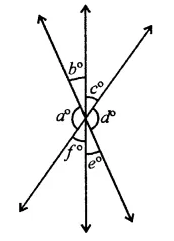In the figure,
(i) a + b = d + c
a° = d°
b° = e°
c°= f°
(ii) a + b + e = 180°
a + e + c = 180°
⇒ a + c + e = 180°
(iii) b + f= e + c
∴ (ii) and (iii) are true statements (d)

Question 10 :
If two interior angles on the same side of a transversal intersecting two parallel lines are in the ratio 2:3, then the measure of the larger angle is
(a) 54°
(b) 120°
(c) 108°
(d) 136°

Answer 10 : In figure, l || m and p is transversal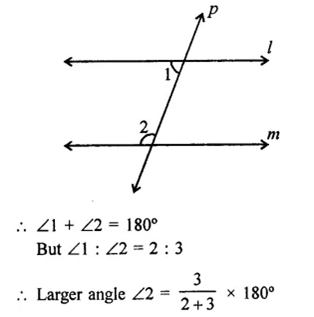=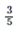x 180° = 108° (c)

Todays Deals### RD Chapter 10- Congruent Triangles Ex-MCQS Contributorskrishan

Name:
Email:

# Latest News# 9000 interview questions in different categories